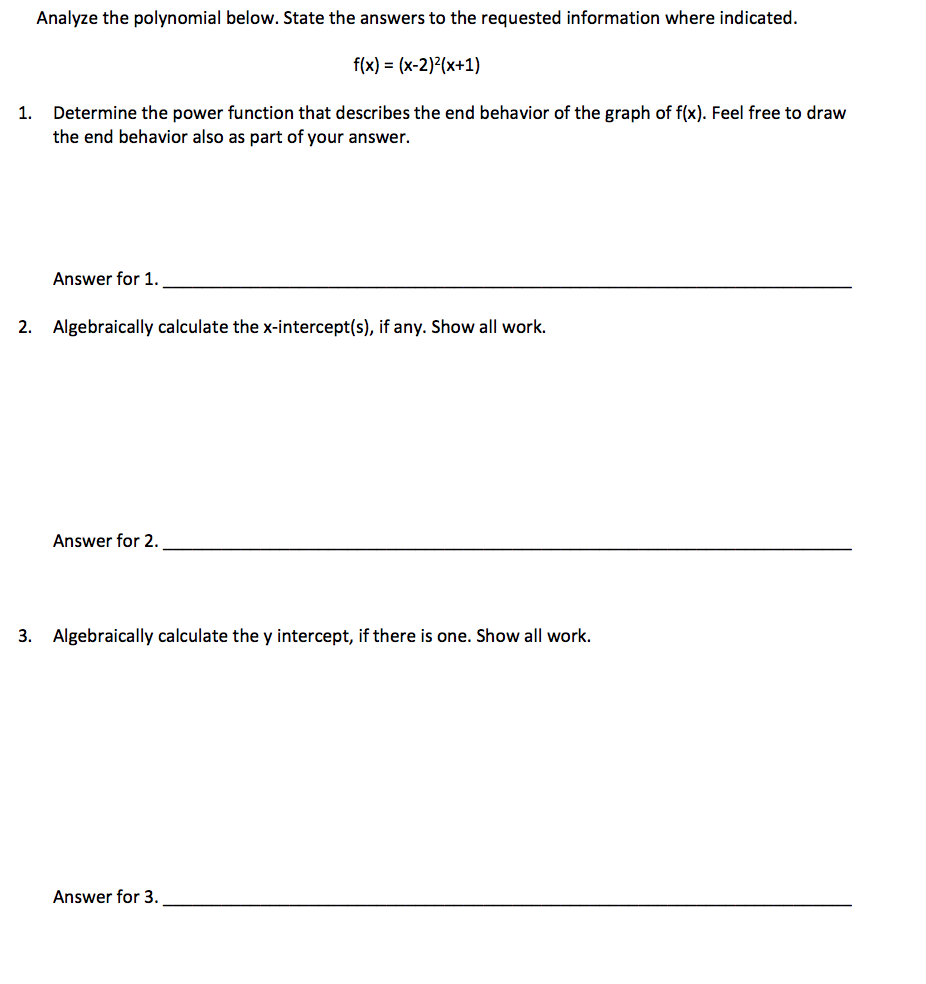# Analyze the polynomial below. State the answers to the requested information where indicated.fx) (x-2x+1)Determine the power function that describes the end behavior of the graph of f(x). Feel free to drawthe end behavior also as part of your answer.1.Answer for 1.2.Algebraically calculate the x-intercept(s), if any. Show all work.Answer for 2.3.Algebraically calculate the y intercept, if there is one. Show all work.Answer for 3.

Question

Can you answer questions from 1 to 3help_outlineImage TranscriptioncloseAnalyze the polynomial below. State the answers to the requested information where indicated. fx) (x-2x+1) Determine the power function that describes the end behavior of the graph of f(x). Feel free to draw the end behavior also as part of your answer. 1. Answer for 1. 2. Algebraically calculate the x-intercept(s), if any. Show all work. Answer for 2. 3. Algebraically calculate the y intercept, if there is one. Show all work. Answer for 3. fullscreen
check_circleExpert Solution
Step 1

1. The power function that describes the behaviour of the given function is the cubic function. For a cubic function the end behaviour is given by,

Step 2

The graph of the given function is as follows.

Step 3
1. Substitute 0 for y to find the x-interce...

### Want to see the full answer?

See Solution

#### Want to see this answer and more?

Solutions are written by subject experts who are available 24/7. Questions are typically answered within 1 hour*

See Solution
*Response times may vary by subject and question
Tagged in

### Polynomials